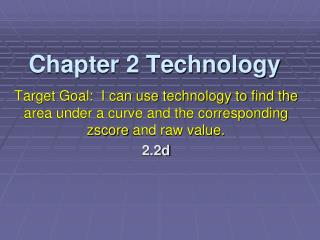DownloadDownload PresentationChapter 2 Technology

# Chapter 2 Technology

Download Presentation## Chapter 2 Technology

- - - - - - - - - - - - - - - - - - - - - - - - - - - E N D - - - - - - - - - - - - - - - - - - - - - - - - - - -
##### Presentation Transcript

1. Chapter 2 Technology Target Goal: I can use technology to find the area under a curve and the corresponding zscore and raw value. 2.2d

2. Finding Areas (plus graph) with “Shadenorm”. Use “shadenorm” to find the area to the left or right of a point without referencing Table A. • Press 2nd VARS(DIST):DRAW • Using exercise 2.45 with N(100,15). We want to find the percent of children whose WISC scores are above 125. • Set WINDOW: X[55,145]15 and Y[-0.008, 0.028].01.

3. “Shadenorm” (You will need to experiment with the y settings to get a good graph. Also, turn off all other graphs; Y= and STAT PLOT.) • Complete the command 2nd VARS(DIST): DRAW: ShadeNorm(125,E99,100,15)

4. “Shadenorm” • To find the area to the left of 85: • Shadenorm(-E99,85,100,15) • When using standard normal values, only specify the end points (mean 0 and standard dev. 1 are understood). • Shadenorm(1,2) finds the area above the interval z = 1 to z = 2.

5. Finding Areas with “normalcdf” It is quicker than shadenorm but does not provide a picture. You do not need to set the Window specs. • Press 2nd VARS(DIST): normalcdf • Complete the command normalcdf (125,E99,100,15). • We can conclude that about 5%of WISC scores are above 125.

6. Finding Areas with “normalcdf” • If normal values have already been standardized ( ie z scores), only specify left and right endpoints. • normalcdf(-1,1) returns 0.6827 • the area from z = -1 to z = 1 is approximately 0.6827 correct to four decimal places.

7. Finding z-values with “invNorm” Calculates the raw or standardized (z score) value corresponding to a known area under a normal distribution or relative frequency. • Press 2nd VARS(DIST):invNorm

8. Complete command invNorm(.9,100,15). • Compare with command invNorm(.9)

9. The first command invNorm(.9,100,15)finds that the rawscore that has 90% of the scores below it from N(100,150) is x = 119. • The second command invNorm(.9) gives the standardized z score, z = 1.28, which has 90% of the scores below it.

10. Exercise 1: Quartiles from a Normal Distribution Find the quartiles for the distribution of blood cholesterol levels for 14-year-old boys. This distribution is N (170 mg/dl, 30mg/dl). • Q1: calculator command? 2ndVARS:invNorm (.25,170,30) = 149.765

11. Finding Quartiles • Q2(M): calculator command? 2ndVARS:invNorm (.50,170,30) = 170 • Q3: calculator command? 2ndVARS:invNorm (.75,170,30) = 190.235